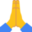# B4J Code SnippetSort and Search Lists in Any Arbitrary Way

In B4X it is easy to sort a List in any arbitrary way, thanks to the fact that we can simply pass the name of a function anywhere.
I am using a non-recursive search here, because I had to sort lists with hundreds of thousands of items. The sorting is quite fast, good enough for sure. B4J is fast!

The name of the function is ListSort, not "SortList", because you may have other ListXxxx utility functions, and this way code completion will show all of them under "List", so it is easier to find them.

This function "lives" in a static code module in my case. It is natural that we must pass the List instance to it, but the second parameter is "Context". Why?

In B4X, you can pass the name of a function to another function, and use it like this:

A function to call:
``````Public Sub CallAFunction(AnObject As Object, FunctionName As String) As TResult
...
CallSub(AnObject, FunctionName)
...
End Sub``````

The AnObject parameter is a class that implements the function. If you want to have a function in something like a static code module, create a class with all the general functions you want to use in the application, create a global instance of it, and use it as if it were a static code module. Call it DelegatedCode, for example.

The ListSort function needs these parameters: the list to sort, a "context" to use with(= the object that has the function AComparer), and the function that compares two items. Then sort the list:

Sort:
``````Public Sub ListSort(lst As List, AContext As Object, AComparer As String) As List
Private ATotal As Int = lst.Size - 1
Private AnOffset As Int = ATotal / 2
Private ALimit As Int
Private ASwitch As Boolean
Private ATmp As Object
Do While AnOffset > 0
ALimit = ATotal - AnOffset
Do While True
ASwitch = False
For i = 0 To ALimit
If CallSub3(AContext, AComparer, lst.Get(i), lst.Get(i + AnOffset)) = ">" Then
ATmp = lst.Get(i)
lst.Set(i, lst.Get(i + AnOffset))
lst.Set(i + AnOffset, ATmp)
ASwitch = True
ALimit = i - AnOffset
End If
Next
If ASwitch = False Then Exit
Loop
AnOffset = AnOffset / 2
Loop
Return lst
End Sub``````

What does the Compare function look like?
The standard Compare functions usually return -1, 0, or 1, when you compare A and B, -1 means A is less that B, 0 means they are equal, 1 means A is greater than B.
In the above sort function, I used "<", "+" and ">" because I found it more intuitive, but you can change it to numbers.

If I have a Type like this:
Type TUser(Age As Int, Name As String)

and I have a List of TUser records, I can have a function to sort them by age first, and then alphabetically, or by name first, and then by age:

SortCompare:
``````Public Function SortCompareAgeFirst(u1 As TUser, u2 As TUser) As String
If u1.Age < u2.age Then Return "<"
If u1.Age > u2.Age Then Return ">"
If u1.Nme < u2.Name Then Return "<"
If u1.AName > u2.Name Then Return ">"
Return "="
End Sub

Public Function SortCompareNameFirst(u1 As TUser, u2 As TUser) As String
If u1.Nme < u2.Name Then Return "<"
If u1.AName > u2.Name Then Return ">"
If u1.Age < u2.age Then Return "<"
If u1.Age > u2.Age Then Return ">"
Return "="
End Sub``````

Then I can sort the list like:

ListSort(AList, DelegatedCode, "SortCompareAgeFirst")

or

ListSort(AList, DelegatedCode, "SortCompareNameFirst")

Now that the list is sorted, we can do binary searches on it. But did we sort it by age first, or by name? You have to remember what you did, because we need another compare function for the search.

It would be natural to pass AKey as TUser in this example, but it is not necessary. For example, I could have an Age and a Name and pass them as an array, and find the TUser in the list with that age and name. The Compare function must be adjusted to this, of course. So in my DelegatedCode object, I have these functions:

SearchCompare:
``````Public Sub SearchCompareAgeFirst(Item1() as Object, Item2 As TUser) As String
If Item1(0).As(Int) < Item2.Age Then Return "<"
If Item1(0).As(String) > Item2.Age Then Return ">"
If Item1(1).As(String) < Item2.Name Then Return "<"
If Item1(1).As(String) > Item2.Name Then Return ">"
Return "="
End Sub

Public Sub SearchCompareNameFirst(Item1() as Object, Item2 As TUser) As String
If Item1(1).As(String) < Item2.Name Then Return "<"
If Item1(1).As(String) > Item2.Name Then Return ">"
If Item1(0).As(Int) < Item2.Age Then Return "<"
If Item1(0).As(String) > Item2.Age Then Return ">"
Return "="
End Sub``````

Then call :

Dim AUser As TUser = ListBinarySearch(AList, DelegatedCode, Array(20, "Joe"), "SearchCompareAgeFirst")

or

Dim AUser As TUser = ListBinarySearch(AList, DelegatedCode, Array(20, "Joe"), "SearchCompareNameFirst")

And the search function is here, based on the post I found on this forum, by Ilan:

Search:
``````'Must be sorted first, see ListSort
Public Sub ListBinarySearch(lst As List, AContext As Object, AKey As Object, AComparer As String) As Object
Dim low As Int
Dim middle As Int
Dim high As Int = lst.Size - 1
Do While low <= high
middle = Floor((low + high) / 2)
Select CallSub3(AContext, AComparer, lst.Get(middle), AKey)
Case "="
Return lst.Get(middle)
Case ">"
high = middle -1
Case Else
low = middle + 1
End Select
Loop
Return Null
End Sub``````

A lot of huffing and puffing for a simple search. I would not do it with just a few objects, like, a few thousand objects. But if you call 100,000 items 1000 times, or if you have some really complex comparison with objects inside objects, you may need it. Just another weapon in the arsenal.

•epiCode